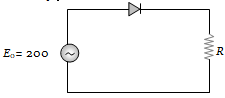# NEET Physics Semiconductor Electronics Questions Solved

A sinusoidal voltage of peak value 200 volt is connected to a diode and resistor R in the circuit shown so that half wave rectification occurs. If the forward resistance of the diode is negligible compared to R the rms voltage (in volt) across R is approximately(a) 200                            (b) 100
(c) $\frac{200}{\sqrt{2}}$                          (d) 280